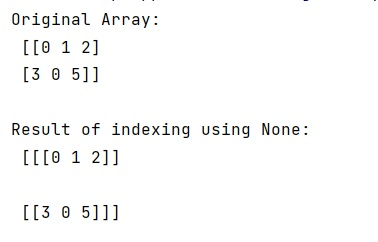# What does selection by [:,None] do in NumPy?

Learn, what is the concept behind indexing in NumPy using [:,None], what does selection by [:,None] do?
Submitted by Pranit Sharma, on February 21, 2023

NumPy is an abbreviated form of Numerical Python. It is used for different types of scientific operations in python. Numpy is a vast library in python which is used for almost every kind of scientific or mathematical operation. It is itself an array which is a collection of various methods and functions for processing the arrays.

## Selection by [:,None]

While indexing in numpy, the newaxis object can be used in all slicing operations to create an axis of length one. The newaxis is an alias for 'None', and 'None' can be used in place of this with the same result.

If we apply indexing on a 1D numpy array using [:,None], it will simply align all the elements in a new axis which means that all the elements in a single row will be aligned in separate rows.

Let us understand with the help of an example,

## Python code to demonstrate the working of selection by [:,None]

```# Import numpy
import numpy as np

# Creating a numpy array
arr = np.array([[0,1,2],[3,0,5]])

# Display original array
print("Original Array:\n",arr,"\n")

# indexing using None
res = arr[:,None]

# Display result
print("Result of indexing using None:\n",res,"\n")
```

Output: